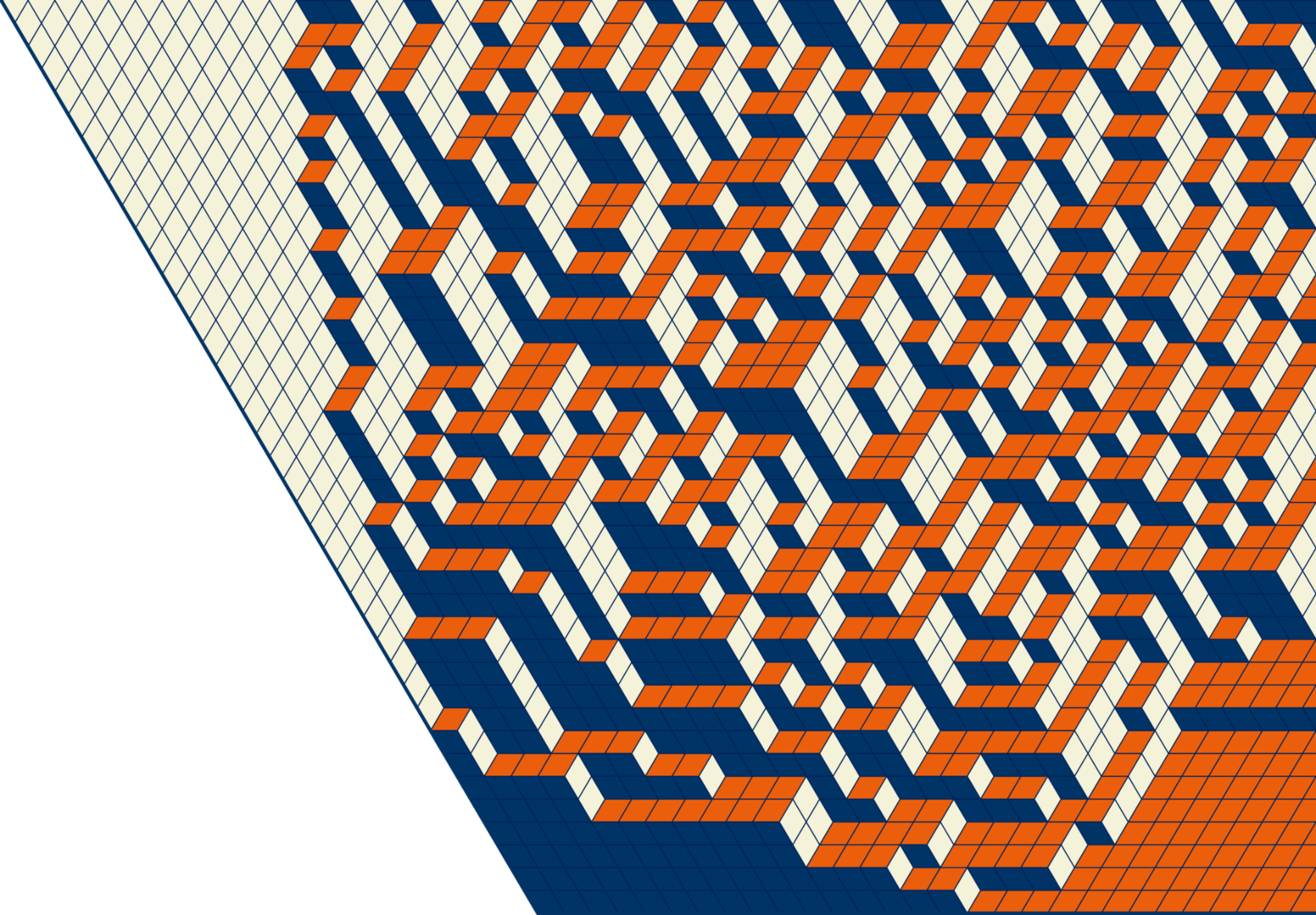Reading seminar on integrable probability in Spring 2019

Reading seminar on integrable probability in Spring 2019

Fridays, 11:00am - 12:30pm, Kerchof 128

We will discuss the classical problem of describing analogues of irreducible representations of the infinite-dimensional unitary group (Edrei-Voiculescu theorem), and its connections with

• representations of finite-dimensional unitary groups
• symmetric functions
• random lozenge tilings and asymptotic combinatorics
• total positivity
• classical analysis (e.g. B-splines)
• von Neumann factor and spherical representations of the infinite-dimensional unitary group
• random matrices

Schedule of meetings

The schedule is subject to change as we progress with the topics.

1. January 18, 2019, special location - Ruffner Hall 127.

Leo Petrov - Introduction “The many facets of the Edrei-Voiculescu theorem”

2. January 25, 2019, special location - Ruffner Hall 123.

Liron Speyer - $U(N)$: weights, irreducible characters, Schur polynomials, branching
[standard textbooks like the H. Weyl’s], a summary is in [section 2.2 in arXiv:1310.8007]

3. February 1, 2019

George Seelinger - Extreme characters of $U(\infty)$: definition and two elementary examples [PDF notes]
[for example, section 4.1.10 in these draft lecture notes]

4. February 8, 2019

Axel Saenz - Functional equation for irreducible characters and the ring theorem for extreme characters of $U(\infty)$
[G. Olshanski, On semigroups related to infinite-dimensional groups. In: Topics in Representation Theory (A. A. Kirillov, ed.). Advances in Soviet Math.] or [A. Borodin, G. Olsahnski “Representations of the Infinite Symmetric Group”, exercises 1.5 and 4.3]

5. February 15, 2019

Ethan Zell - Projection formula for Gibbs measures on tilings (formula for the number of trapezoidal Gelfand-Tsetlin schemes)
[arXiv:1109.1412 and arXiv:1208.3443]

6. February 22, 2019

Leo Petrov - Idea of proof of Edrei-Voiculescu theorem
[arXiv:1109.1412]

7. March 1, 2019

No meeting

8. March 8, 2019

Joseph Eisner - Characters and von Neumman factor representations. GNS construction

9. March 22, 2019

Axel Saenz - Pascal triangle as a branching graph [PDF notes]
[Kerov, “Combinatorial examples in the theory of AF-algebras”], [A. Borodin, G. Olsahnski “Representations of the Infinite Symmetric Group”], these draft lecture notes

10. March 29, 2019

Arun Kannan - Diffusions from dynamics on the Pascal triangle
[arXiv:0706.1034, section 2]

11. April 5, 2019

No meeting

12. April 12, 2019

George Seelinger - A q-analogue of de Finetti’s theorem
[arXiv:0905.0367]

13. April 19, 2019

Liron Speyer - Young graph and its boundary
[A. Borodin, G. Olsahnski “Representations of the Infinite Symmetric Group”]

14. April 26, 2019

Joseph Eisner - Construction of extreme representations of the infinite symmetric group
Chapter 10, [A. Borodin, G. Olsahnski “Representations of the Infinite Symmetric Group”]A fragment of a random heart